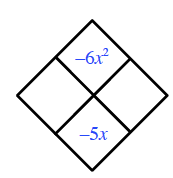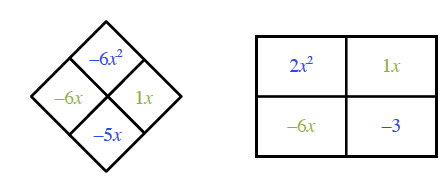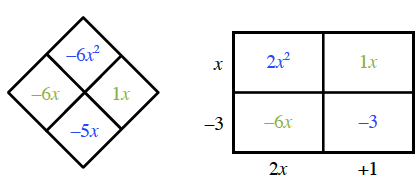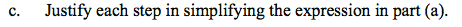Home > CCA2 > Chapter 10 > Lesson 10.2.1 > Problem10-93

10-93.
1. Factor and simplify each expression below. Homework Help ✎

1.2.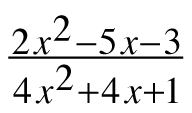3. Justify each step in simplifying the expression in part (a).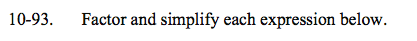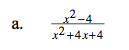Factor and then reduce.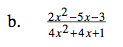For the numerator, what numbers multiply to −6 and add to −5?

Put these numbers in a generic rectangle (these create your "middle term") along with the first and last terms.
Now find the greatest common factors of each row and column.

See the generic rectangle.
2x2 − 5x − 3 = (x − 3)(2x + 1)

Now factor the denominator and simplify.